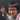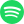# My (Overcomplicated) Coherent System of Measurements

July 23, 2020 · 18:25 pm · Metrology

There are many measurement systems, and the ones by Système international and the imperial system are arguably two of the most famous. There are also the furlong-firkin-fortnight (FFF) system and the CCC system by Jan Misali, both of which are sarcastic systems made as a joke. I have here a system that falls into the latter category that aims to be as difficult to use as possible. Here are the base units:

UnitDefinition
Barye $(Ba)$$0.1 Pa = 0.1 \frac{kg}{m \cdot s^2}$
gallon $(gal)$$231 in^3 = 0.003785411784 m^3$
standard gravity $(g)$$9.80665 \frac{m}{s^2}$
vacuum permittivity $(\epsilon_0)$$\approx 8.8541878128 \times 10^{-12} \frac{C^2 \cdot s^2}{kg \cdot m^3}$1
specific heat of water at 25°C $(c)$$\approx 4179.6 \frac{m^2}{s^2 \cdot K}$1

Let’s try to derive the units for some common dimensions.

## Length

We know volume is represented by $gal$, so $\sqrt{gal} \approx 0.1558491279 m$ is our unit of length.

## Time

We can’t use $Ba$ nor $\epsilon_0$, since those have $kg$ and $C$, respectively, that needs to be removed by multiplying its own reciprocal, which cancels itself out. That leaves us with $gal$ and $g$ to be manipulated. Time can be represented by seconds, so we have

\begin{aligned} s &= \sqrt{s^2} \\ &= \sqrt{\frac{s^2}{m} \cdot m} \\ &= \sqrt{\frac{s^2}{m} \sqrt{m^3}} \\ &= \sqrt{\frac{s^2}{m}} \sqrt{m^3} \\ \end{aligned}

We can replace $\frac{s^2}{m}$ with $g^{-1}$ and $m^3$ with $gal$ to get

$\sqrt{g^{-1}} \sqrt{gal} \approx 0.1260642227 s.$

## Velocity

Velocity is acceleration times time, so

\begin{aligned} g \cdot \sqrt{g^{-1}} \sqrt{gal} &= \sqrt{g} \sqrt{gal} \\ &\approx 1.2362677099 \frac{m}{s} \\ \end{aligned}

## Mass

\begin{aligned} kg &= \frac{kg}{m \cdot s^2} m \cdot s^2 \\ &= \frac{kg}{m \cdot s^2} m^2 \cdot \frac{s^2}{m} \\ \end{aligned}

We can replace $\frac{kg}{m \cdot s^2}$ and $m^2$ with $Ba$ and $\sqrt{gal^2}$, respectively, to get

$Ba \cdot g^{-1} \sqrt{gal^2} \approx 2.4767836812 \times 10^{-4} kg$

## Force

Force is mass times acceleration, so

\begin{aligned} Ba \cdot g^{-1} \sqrt{gal^2} \cdot g &= Ba \sqrt{gal^2} \\ &\approx 0.002428895068 N \\ \end{aligned}

## Momentum

Momentum is mass times velocity, so

\begin{aligned} Ba \cdot g^{-1} \sqrt{gal^2} \sqrt{g} \sqrt{gal} &= Ba \sqrt{g^{-1}} \sqrt{gal^5} \\ &\approx 3.06196768983 \times 10^{-4} \frac{kg \cdot m}{s} \\ \end{aligned}

## Energy

Energy has the same units as work, which is force times distance, so

\begin{aligned} Ba \sqrt{gal^2} \cdot \sqrt{gal} &= Ba \cdot gal \\ &\approx 3.785411784 \times 10^{-4} N \cdot m \\ \end{aligned}

## Electric Charge

\begin{aligned} C &= \sqrt{C^2} \\ &= \sqrt{\frac{C^2 \cdot s^2}{kg \cdot m^3} \cdot \frac{kg \cdot m^3}{s^2}} \\ &= \sqrt{\frac{C^2 \cdot s^2}{kg \cdot m^3} \cdot \frac{kg \cdot m}{s} \cdot \frac{m}{s} \cdot m} \\ \end{aligned}

We can replace in $\epsilon_0$, momentum, velocity, and length accordingly:

\begin{aligned} \sqrt{\epsilon_0 \cdot Ba \sqrt{g^{-1}} \sqrt{gal^5} \cdot \sqrt{g} \sqrt{gal} \cdot \sqrt{gal}} &= \sqrt{\epsilon_0 \cdot Ba \sqrt{gal^5} \cdot \sqrt{gal} \cdot \sqrt{gal}} \\ &= \sqrt{\epsilon_0 \cdot Ba \cdot \sqrt{gal^4}} \\ &= \sqrt{\epsilon_0 \cdot Ba} \sqrt{gal^2} \\ &\approx 2.285509959336463587506 \times 10^{-8} C\\ \end{aligned}

## Electric Field

Electric Field is force per charge, so

\begin{aligned} \frac{Ba \sqrt{gal^2}}{\sqrt{\epsilon_0 \cdot Ba} \sqrt{gal^2}} &= \sqrt{\frac{Ba}{\epsilon_0}} \\ &\approx 106273.65935983474 \frac{N}{C}\\ \end{aligned}

## Voltage

Voltage is energy per charge, so

\begin{aligned} \frac{Ba \cdot gal}{\sqrt{\epsilon_0 \cdot Ba} \sqrt{gal^2}} &= \sqrt{\frac{Ba}{\epsilon_0}} \sqrt{gal} \\ &\approx 16562.657137136224 V \\ \end{aligned}

## Capacitance

Capacitance is charge per voltage, so

\begin{aligned} \frac{\sqrt{\epsilon_0 \cdot Ba} \sqrt{gal^2}}{\sqrt{\frac{Ba}{\epsilon_0}} \sqrt{gal}} &= \epsilon_0 \sqrt{gal} \\ &\approx 1.37991744948 \times 10^{-12} F \\ \end{aligned}

## Current

Current is charge per time, so

\begin{aligned} \frac{\sqrt{\epsilon_0 \cdot Ba} \sqrt{gal^2}}{\sqrt{g^{-1}} \sqrt{gal}} &= \sqrt{\epsilon_0 \cdot Ba \cdot gal \cdot g} \\ &\approx 1.81297271306 \times 10^{-7} A \\ \end{aligned}

## Resistance

Resistance has the same units as vacuum permittivity divided by velocity, so

$\frac{\epsilon_0}{\sqrt{g} \sqrt{gal}} \approx 7.1620311209 \times 10^{-12} \Omega$

## Temperature

\begin{aligned} K &= \frac{s^2 \cdot K}{m^2} \cdot \frac{m^2}{s^2} \\ \end{aligned}

We can replace in $c$ and velocity accordingly:

$c^{-1} \sqrt{g} \sqrt{gal} \approx 2.95786130251 \times 10^{-4} K$

## What about luminous intensity and substance amount?

I don’t know how luminous intensity works so I can’t list an overcomplicated version of it. But feel free to use candela.

For substance amount, I don’t know any established units that are in the range of Avogadro’s Number that are ridicuous, so I’ll just say the Baker’s Dozen $(13)$ works.

1. Technically, these don’t have a defined exact value, but I couldn’t think of any other unit or constant that was as complex.Streaming direct thought dumps from Yuto Nishida.
Connect with me on LinkedIn! I'm currently listening to: